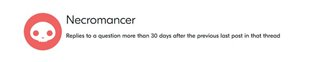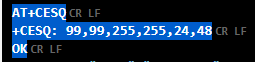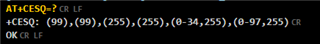# How know RSSI?

Hi

I need to know the RSSI but I don´t know how do that, I have a nRF9160DK kit with onboard gps antenna and I have proved the gps sample but it don't show information about power of signal.

I have another doubt, what is the period of time where I can do a question without be a necromancer?

Thanks

Julio

Parents
• Hi,

I need to know the RSSI

LTE:

There are no way to get the RSSI directly when using the nRF9160. However, you can use the +CESQ AT command to get the RSRP and RSRQ.

You can then calculate the RSSI like this:

RSSI = 10log(N) + RSRP - RSRQ.

N is Resource Blocks and is 6 for LTE M1.

GPS:

Again, it is not possible to get the RSSI directly. Instead, you get the CN0, which is the carrier-to-noise-density ratio (or the ratio of the signal level to the (noise level in 1Hz BW)).

If you know the Noise Figure of the receiver, the RSSI = -174dBm + NF + CN0.

I have another doubt, what is the period of time where I can do a question without be a necromancer?

I assume you are talking about the Necromancer achievement?

In that case, it is 30 days:Best regards,

Didrik

Reply
• Hi,

I need to know the RSSI

LTE:

There are no way to get the RSSI directly when using the nRF9160. However, you can use the +CESQ AT command to get the RSRP and RSRQ.

You can then calculate the RSSI like this:

RSSI = 10log(N) + RSRP - RSRQ.

N is Resource Blocks and is 6 for LTE M1.

GPS:

Again, it is not possible to get the RSSI directly. Instead, you get the CN0, which is the carrier-to-noise-density ratio (or the ratio of the signal level to the (noise level in 1Hz BW)).

If you know the Noise Figure of the receiver, the RSSI = -174dBm + NF + CN0.

I have another doubt, what is the period of time where I can do a question without be a necromancer?

I assume you are talking about the Necromancer achievement?

In that case, it is 30 days:Best regards,

Didrik

Children
• Hi Didrik

I have proved +CESQ AT and get these values and used the equation, is result in dBm?

10*LOG(6)+48-24 = 31.78also I tried +CESQ AT=? command with follow results, but I don't know how to interpret these expression (0-34,255) and (0-97,255)• tracking said:
also I tried +CESQ AT=? command with follow results, but I don't know how to interpret these expression (0-34,255) and (0-97,255)

The test command (=?) lists the ranges the values can have. I.e. the RSRQ can be between 0 and 34, or 255 (which indicates that it is unknown/no valid). The RSRP can be between 0 and 97, or 255.

https://infocenter.nordicsemi.com/topic/ref_at_commands/REF/at_commands/mob_termination_ctrl_status/cesq_test.html

tracking said:
I have proved +CESQ AT and get these values and used the equation, is result in dBm?

Yes.

But, to the values you get from +CESQ are not the RSRQ and RSRP directly.

To get the RSRQ, you must subtract 19.5, and to get the RSRP you must subtract 140.

In your case, the result will be 10*log(6) + (48 - 140) - (24 - 19.5) = 10*log(6) + (-92) - 4.5 = -88.7dBm

Related